# [Paper] Random Erasing (RE): Random Erasing Data Augmentation (Image Classification)

## Improves Models for Image Classification, Object Detection & Person Re-identificationRandom Erasing (From Author’s GitHub: https://github.com/zhunzhong07/Random-Erasing) Meow!

In this story, Random Erasing Data Augmentation (Random Erasing, RE), by Xiamen University, University of Technology Sydney, Australian National University, and Carnegie Mellon University, is shortly presented. In this paper:

• Random Erasing is proposed to randomly select a rectangle region in an image and erases its pixels with random values.
• This reduces the risk of overfitting and makes the model robust to occlusion.
• It is is complementary to commonly used data augmentation techniques such as random cropping and flipping.

This is a paper in 2020 AAAI with over 600 citations. (Sik-Ho Tsang @ Medium)

# Outline

1. Random Erasing (RE)
2. Ablation Study
3. Experimental Results

# 1. Random Erasing (RE)

## 1.1. Random Erasing (RE) Algorithm

• For an image I in a mini-batch, the probability of it undergoing Random Erasing is p.
• Random Erasing randomly selects a rectangle region Ie in an image, and erases its pixels with random values.
• The area of the image is S = W ×H. The area of erasing rectangle region is randomized as Se, where Se/S is in range specified by minimum sl and maximum sh.
• The aspect ratio re of erasing rectangle region is randomly initialized between r1 and r2.
• The size of Ie is:
• and:
• If xe+We W and ye+HeH, we set the region, Ie = (xe, ye, xe +We, ye +He), as the selected rectangle region.
• With the selected erasing region Ie, each pixel in Ie is assigned to a random value in [0, 255].
• The detailed RE algorithm is as below:

## 1.2. Random Erasing for Image Classification and Person Re-identification

• In general, training data does not provide the location of the object. In this case, Random Erasing is performed on the whole image.

## 1.3. Random Erasing for Object Detection

• There are 3 schemes.
1. Image-aware Random Erasing (IRE): selecting erasing region on the whole image.
2. Object-aware Random Erasing (ORE): selecting erasing regions in the bounding box of each object. if there are multiple objects in the image, Random Erasing is applied on each object separately.
3. Image and object-aware Random Erasing (I+ORE): selecting erasing regions in both the whole image and each object bounding box.

## 1.4. Comparison with Random Cropping

• Random cropping reduces the contribution of the background.
• CNN can learn the model on the presence of parts of the object instead of focusing on the whole object.
• Random Erasing retains the overall structure of the object, only occluding some parts of object. Areas are re-assigned with random values, which can be viewed as adding noise to the image.
• They can be complementary to each other.

# 2. Ablation Study

## 2.1. The impact of hyper-parameters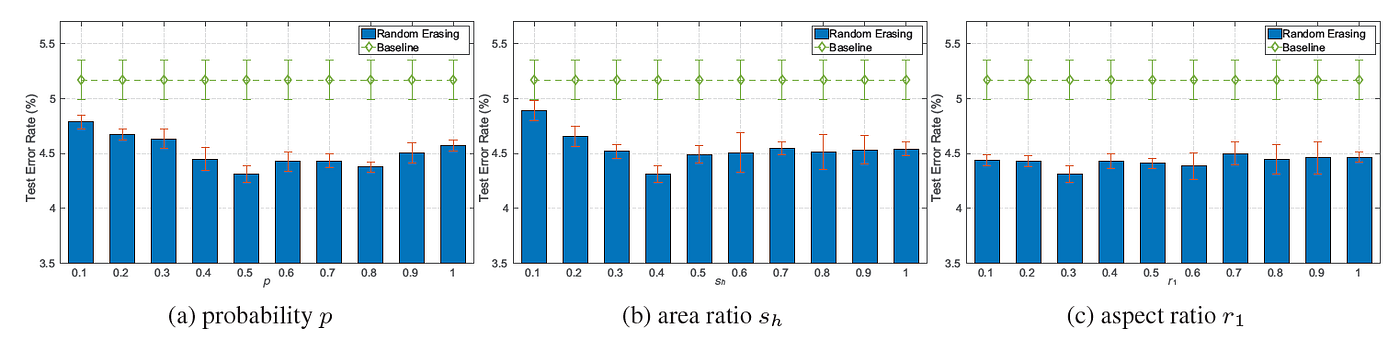Test errors (%) under different hyper-parameters on CIFAR-10 with using Pre-Activation ResNet-18
• Pre-Activation ResNet-18 is used as baseline.
• 3 hyperparameters to evaluate, i.e., the erasing probability p, the area ratio range of erasing region sl and sh, and the aspect ratio range of erasing region r1 and r2.
• To simplify experiment, sl is fixed to 0.02, r1 = 1/r2 and evaluate p, sh, and r1.
• p = 0.5, sh = 0.4 and r1 = 0.3 as the base setting, and alter one of them.
• When p ∈ [0.2, 0.8] and sh ∈ [0.2, 0.8], the average classification error rate is 4.48%, outperforming the baseline method (5.17%) by a large margin.
• For aspect ratio, the best result are obtained when r1 = 0.3, error rate = 4.31%, reduces the classification error rate by 0.86% compared with the baseline.
• p = 0.5, sl = 0.02, sh = 0.4, and r1 = 1/r2 = 0.3 as default settings.

## 2.2. Four Types of Random Values

1. RE-R: Random value ranging in [0, 255].
2. RE-M: mean ImageNet value.
3. RE-0: 0.
4. RE-255: 255.
• RE-R achieves approximately equal performance to RE-M, RE-R is chosen.

# 3. Experimental Results

## 3.1. Image Classification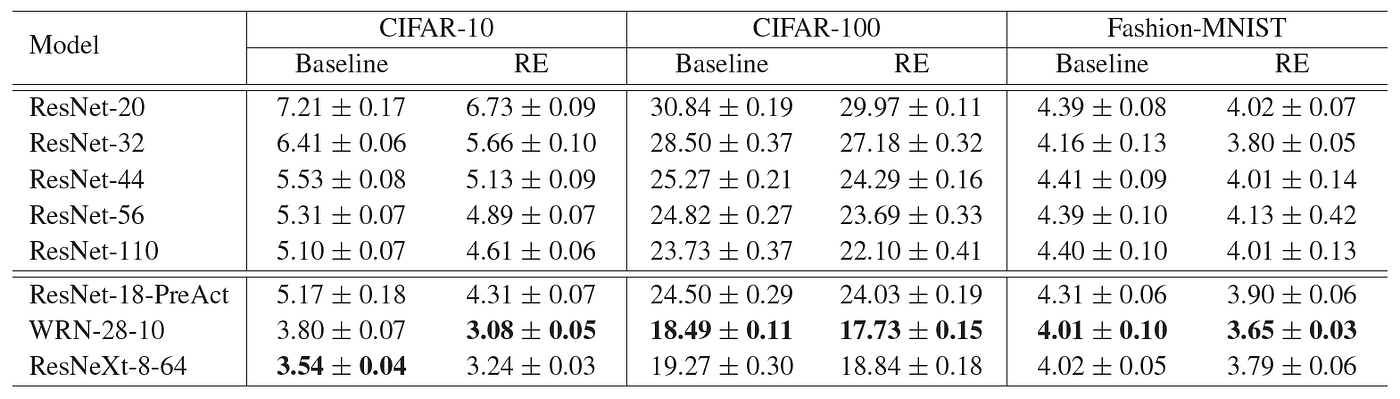Test errors (%) with different architectures on CIFAR-10, CIFAR-100 and Fashion-MNIST
• p = 0.5, sl = 0.02, sh = 0.4, and r1 = 1/r2 = 0.3.
• For CIFAR-10, random erasing improves the accuracy by 0.49% using ResNet-110.
• Random erasing obtains 3.08% error rate using WRN-28–10, which improves the accuracy by 0.72%.
• For CIFAR-100, random erasing obtains 17.73% error rate which gains 0.76% than the WRN-28-10 baseline.
• Random erasing improves WRN-28–10 from 4.01% to 3.65% in top-1 error on Fashion-MNIST.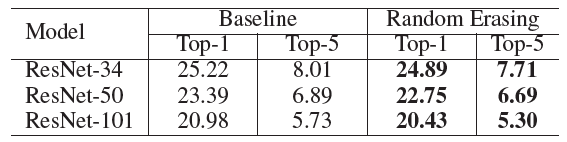Test errors (%) on ImageNet-2012 validation set
• Random erasing consistently improves the results on all three ResNet variants on ImageNet.

## 3.2. Comparison with Dropout and Random Noise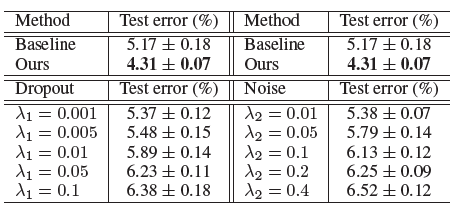Test errors (%) with different data augmentation methods on CIFAR-10
• Applying Dropout or adding random noise at the image layer fails to improve the accuracy.

## 3.3. Comparing with Data Augmentation Methods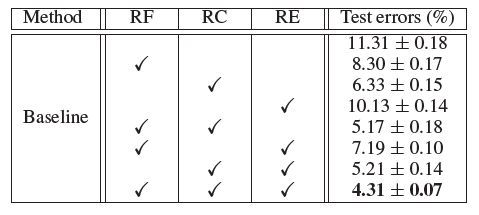Test errors (%) with different data augmentation methods on CIFAR-10
• RF: Random flipping, RC: Random cropping, RE: Random Erasing.
• Random Erasing and the two competing techniques are complementary. Particularly, combining these three methods achieves 4.31% error rate, a 7% improvement over the baseline without any augmentation.

## 3.4. Robustness to Occlusion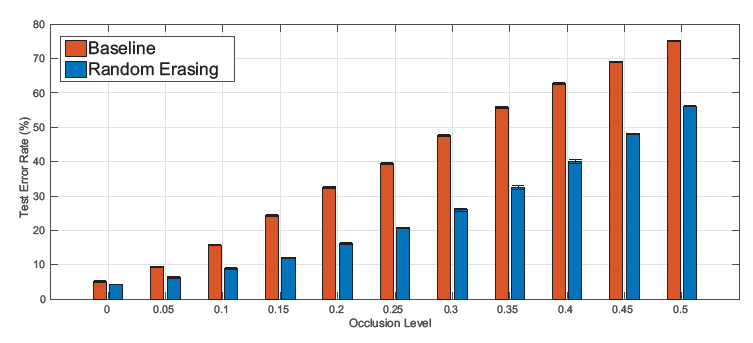Test Error Rates Against Different Occlusion Levels
• The baseline performance drops quickly when increasing the occlusion level l.
• Random erasing approach achieves 56.36% error rate when the occluded area is half of the image (l = 0.5), while the baseline rapidly drops to 75.04%.
• Random Erasing improves the robustness of CNNs against occlusion.

## 3.5. Object Detection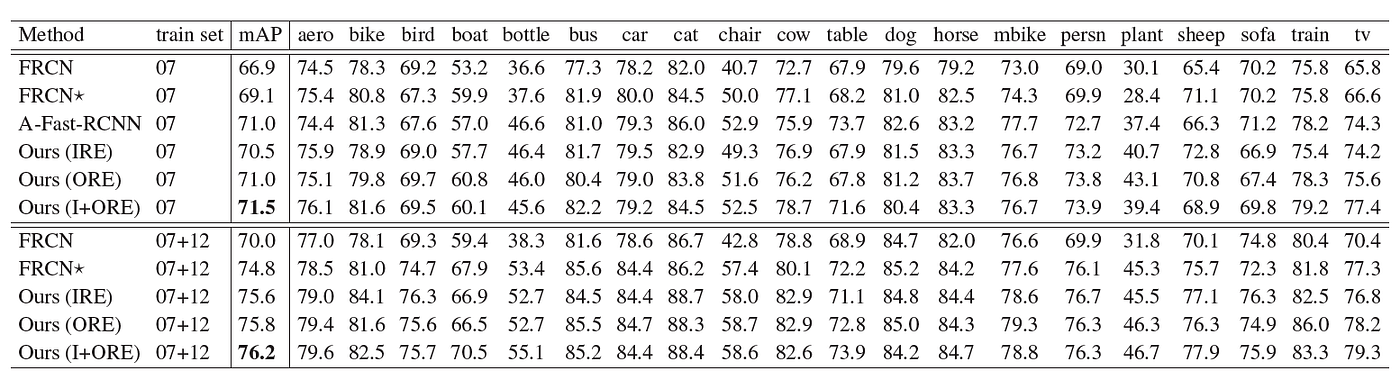VOC 2007 test detection average precision (%)
• Faster R-CNN using VGG-16 backbone is used as baseline.
• For Random Erasing, p = 0.5, sl = 0.02, sh = 0.2, and r1 = 1/r2=0.3.
• The baseline got 69.1% mAP.
• The detector training with I+ORE obtains further improved in performance with 71.5% mAP.
• When using the enlarged 07+12 training set, 76.2% mAP is achieved.

## 3.6. Person Re-identification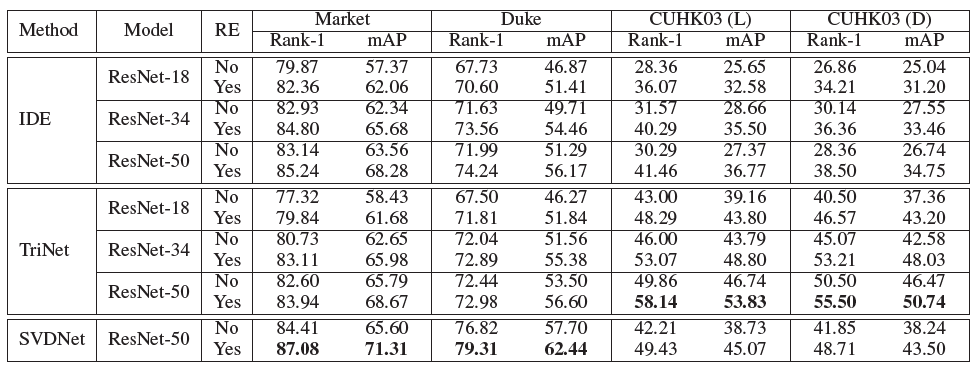Person re-identification performance with Random Erasing (RE) on Market-1501, DukeMTMC-reID, and CUHK03
• For Random Erasing, p = 0.5, sl = 0.02, sh = 0.2, and r1 = 1/r2=0.3.
• For Market-1501, Random Erasing improves the rank-1 by 3.10% and 2.67% for IDE and SVDNet with using ResNet-50.
• For DukeMTMC-reID, Random Erasing increases the rank-1 accuracy from 71.99% to 74.24% for IDE (ResNet-50) and from 76.82% to 79.31% for SVDNet (ResNet-50).
• For CUHK03, TriNet gains 8.28% and 5.0% in rank-1 accuracy when applying Random Erasing.
• This indicates that Random erasing can reduce the risk of over-fitting and improves the re-ID performance.

--

--